# Multi-layer Neural Network.¶

By virture of being here, it is assumed that you have gone through the Quick Start. Let us take this one step further and create a neural network with two hidden layers. We begin as usual by importing the network class and creating the input layer.

from yann.network import network
net = network()
dataset_params  = { "dataset": "_datasets/_dataset_xxxxxx", "id": 'mnist', "n_classes" : 10 }
net.add_layer(type = "input", id ="input", dataset_init_args = dataset_params)


Instead of connecting this to a classfier as we saw in the Quick Start , let us add a couple of fully connected hidden layers. Hidden layers can be created using layer type = dot_product.

net.add_layer (type = "dot_product",
origin ="input",
id = "dot_product_1",
num_neurons = 800,
regularize = True,
activation ='relu')

origin ="dot_product_1",
id = "dot_product_2",
num_neurons = 800,
regularize = True,
activation ='relu')


Notice the parameters passed. num_neurons is the number of nodes in the layer. Notice also how we modularized the layers by using the id parameter. origin represents which layer will be the input to the new layer. By default yann assumes all layers are input serially and chooses the last added layer to be the input. Using origin, one can create various types of architectures. Infact any directed acyclic graphs (DAGs) that could be hand-drawn could be implemented. Let us now add a classifier and an objective layer to this.

net.add_layer ( type = "classifier",
id = "softmax",
origin = "dot_product_2",
num_classes = 10,
activation = 'softmax',
)

id = "nll",
origin = "softmax",
)


Again notice that we have supplied a lot more arguments than before. Refer the API for more details. Let us create our own optimizer module this time instead of using the yann default. For any module in yann, the initialization can be done using the add_module method. The add_module method typically takes input type which in this case is optimizer and a set of intitliazation parameters which in our case is params = optimizer_params. Any module params, which in this case is the optimizer_params is a dictionary of relevant options. A typical optimizer setup is:

optimizer_params =  {
"momentum_type"       : 'polyak',
"momentum_params"     : (0.9, 0.95, 30),
"regularization"      : (0.0001, 0.0002),
"optimizer_type"      : 'rmsprop',
"id"                  : 'polyak-rms'
}
net.add_module ( type = 'optimizer', params = optimizer_params )


We have now successfully added a Polyak momentum with RmsProp back propagation with some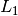and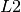co-efficients that will be applied to the layers for which we passed as argument regularize = True. For more options of parameters on optimizer refer to the optimizer documentation . This optimizer will therefore solve the following error: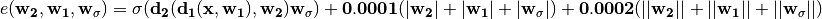where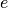is the error,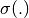is the sigmoid layer and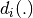is the ith layer of the network. Once we are done, we can cook, train and test as usual:

learning_rates = (0.05, 0.01, 0.001)

net.cook( optimizer = 'polyak-rms',
objective_layer = 'nll',
datastream = 'mnist',
classifier = 'softmax',
)

net.train( epochs = (20, 20),
validate_after_epochs = 2,
training_accuracy = True,
learning_rates = learning_rates,
show_progress = True,
early_terminate = True)


The learning_rate, supplied here is a tuple. The first indicates a annealing of a linear rate, the second is the initial learning rate of the first era, and the third value is the leanring rate of the second era. Accordingly, epochs takes in a tuple with number of epochs for each era.

This time, let us not let it run the forty epochs, let us cancel in the middle after some epochs by hitting ^c. Once it stops lets immediately test and demonstrate that the net retains the parameters as updated as possible. Once done, lets run net.test().

Some new arguments are introduced here and they are for the most part easy to understand in context. epoch represents a tuple which is the number of epochs of training and number of epochs of fine tuning epochs after that. There could be several of these stages of finer tuning. Yann uses the term ‘era’ to represent each set of epochs running with one learning rate. show_progress will print a progress bar for each epoch. validate_after_epochs will perform validation after such many epochs on a different validation dataset. The full code for this tutorial with additional commentary can be found in the file pantry.tutorials.mlp.py. If you have toolbox cloned or downloaded or just the tutorials downloaded, Run the code as,

from pantry.tutorials.mlp import mlp
mlp(dataset = 'some dataset created')


or simply,

python pantry/tutorials/mlp.py


from the toolbox root or path added to toolbox. The __init__ program has all the required tools to create or load an already created dataset. Optionally as command line argument you can provide the location to the dataset.

pantry.tutorials.mlp.mlp(dataset, verbose=1)[source]

This method is a tutorial on building a two layer multi-layer neural network. The built network is mnist->800->800->10 .It optimizes with polyak momentum and rmsprop.

Parameters: dataset – an already created dataset.